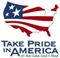Scientific Investigations Report 2008–5093

U.S. GEOLOGICAL SURVEY
Scientific Investigations Report 2008–5093

Table 8. Grain shear stress, largest mobilized particle, and particle classification for a factor of 0.5, 1.0, and 1.5 times the dune height and (or) dune length for simulation 5 (river discharge, 28,900 cubic feet per second), Coeur d’Alene River near Dudley, Idaho.

[Abbreviations: da, largest mobilized particle size in millimeters; N/m2, Newtons per square meter; ft, feet; mm, millimeter. Symbols: λ, wave length of dune, in feet; ∆, wave height of dune in feet; τb, FASTMECH simulated bed shear stress, in Newtons per square meter (see equation 3); τ’o, grain shear stress, in Newtons per square meter (see equation 5)]

Model simulated results
Average τb = 5.7 N/m2
Maximum τb = 6.7 N/m2
Wave length (λ) multiplication factor
0.5
(λ = 31.5 ft)
1.0
(λ = 63 ft)
1.5
(λ = 94.5 ft)
Wave
height (∆)
multiplication
factor
0.5
(∆ = 0.55 ft)
τ’o = 3.7 N/m2
da = 5.3 mm
Fine gravel
τ’o = 4.5 N/m2
da = 6.4 mm
Fine gravel
τ’o = 4.9 N/m2
da = 6.9 mm
Fine gravel
1.0
(∆ = 1.1 ft)
τ’o = 2.5 N/m2
da = 3.0 mm
Very fine gravel
τ’o = 3.5 N/m2
da = 5.1 mm
Fine gravel
τ’o = 4.0 N/m2
da = 5.7 mm
Fine gravel
1.5
(∆ = 1.65 ft)
τ’o = 1.8 N/m2
da = 2.8 mm
Very fine gravel
τ’o = 2.8 N/m2
da = 4.1 mm
Fine gravel
τ’o = 3.4 N/m2
da = 4.9 mm
Fine gravelU.S. Department of the Interior | U.S. Geological Survey